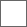• 38回复贴，共1

### 武烈皇后获取的大概推测

20+252+110+15=397
397×17.5=6947.5
2100÷17.5=120

3×14×0.75=31.5个红包，65×14=910元宝，

120-50=70，70÷4=17.5个红包777武将是只要稍微活跃点肯定能拿到的